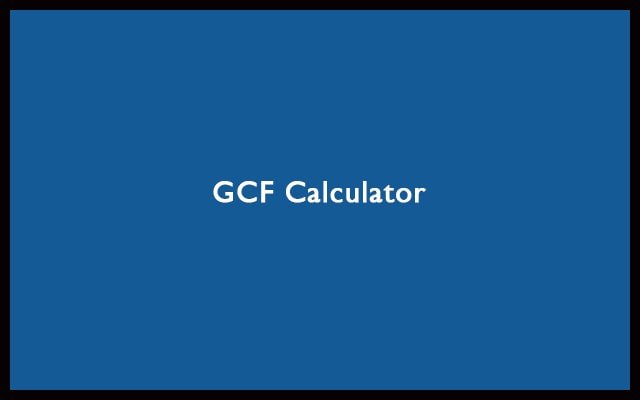# GCF Calculator – Greatest Common Factor Calculator

Sometimes we need to solve the greatest common factor among some numbers while doing mathematical calculations. Therefore, use this GCF Calculator to find the GCF and LCM. The greatest common factor is known as GCF. You can find the GCF of numbers easily using this calculator. It’s free and simple to use.

 First Number: Second Number: Greatest Common Factor (GCF): Least Common Multiple (LCM):
Related### What is Greatest Common Factor?

There might be a query in your mind that what’s GCF? First of all, the greatest common factor, highest common factor and greatest common divisor are all the same things. The method of all these is identical. Furthermore, they convey the same thing. What’s that thing? The greatest factor common between the numbers. The highest number is divisible along with zero remainders. In this article, we will discuss more on it. Also, after looking at some of the examples. You will be more confident about this stuff. You will also get to know about the working of the GCF calculator and the mathematics behind it.

#### Factors Calculation

To determine the Greatest common factor. You need to first find out the factors. Factors are the whole numbers. Which when divided with the number gives the remainder zero. If we multiply all the factors, the resulting number will be the number whose factors have been written. Use the Least common factor technique. It’s an easy way to find factors.

For example, the factors of 10 are 1, 2, and 5. Since, 10 is evenly divisible by 1, 2, 5, and 10. In all cases, it remains remainder zero. Also, if we multiply them, we will get 10. Hence, we can say that. 10 = 1 x 2 x 5 Or 1 x 10 = 10. That’s how we write the factors for a number.

For prime numbers, there will be only two factors. Since prime numbers are only evenly divisible by 1 and themselves. Some examples of prime numbers are 2,3,5 etc. Whereas, for composite numbers, there will be more than two factors. You can use the GCF calculator to find it. The greatest common factor calculator is the best tool to know the factors of prime and composite numbers. Let’s look over some examples. To understand the better concept of determining factors.

For example:

Find the factors of 15, 20, and 25.

Solution:

Factors of

15 = 1, 3, 5 and 15
20 = 1, 2, 4, 5, 10 and 20
25 = 1 and 25

## How GCF Calculator Works?

After knowing how to find factors. Then using the greatest common factors. We will determine the GCF. You should also know about prime factorization. All the factors should be prime numbers. Then select all the common prime factors. The product of it gives the Greatest common factor. This is how the GCF calculator works.

For Example:

##### What is the GCF of 5 and 10?

Prime factors of 5 are 1 x 5
The prime factors of 10 are 1 x 2 x 5

The common prime factor is 1 and 5. Hence, the GCF is also 5.

##### What is the GCF of 6 and 14?

Prime factors of 6 are 1 x 2 x 3.
The prime factors of 14 are 1 x 2 x 7.

The common prime factor is 1 and 2. Hence, the GF is 2.

##### What is the GCF of 10, 18 and 26?

The prime factors of

10 = 1 x 2 x 5
18 = 1 x 2 x 3 x 3
26 = 1 x 2 x 13

The common factor is 1 and 2. So, the GCF is 2.

There is another way of finding the Greatest Common Factor. That’s Euclid’s Algorithm. It is an easy way to determine GCF. However, by using the GCF Calculator or Greatest Common Factor Calculator you will not find difficulties.

#### Why to go for the GCF Calculator?

There is no device calculator for finding GCF. So, use our greatest common factor calculator. It has many advantages. Like accuracy. Also, it saves time. You can find results in seconds. Manual calculations take much more time. As compared to using the tool. You also get 100% accurate. Now, just open the browser. Open the GCF Calculator. Then enter the numbers to find their GCF.